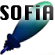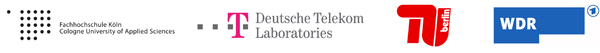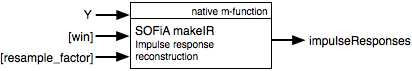Welcome to SOFiA
Who is behind SOFiA
Feature overview
System overview
Function reference
mergeArrayData
F/D/T
gauss
lebedev
S/W/G
S/T/C
W/G/C
S/F/E
M/F
R/F/I
P/D/C
I/T/C
makeMTX
makeIR
visual3D
Coordinate System
Application Examples
Example 1
Example 2
Example 3
Example 4
Example 5
Example 6
Example 7
Example 8
Array Datasets
VariSphear system
Groups and Mailinglists
Contact and Support
How to Reference

# SOFiA Impulse Response Reconstruction

This function recombines impulse responses or time domain signals for multiple channels from frequency domain data delivered by P/D/C or I/T/C. The internal IFFT blocklength is determined by the Y data itself: Y should have a size of [NumberOfChannels x ((2^n)/2)+1] with n={1, 2 ,3 , ...} (which is the case when using the SOFiA readVSAdata() or mergeArrayData() functions for data import) and the function returns [NumberOfChannels x resampleFactor*2^n samples. The impulse responses are windowed with a HANN window. The argument win can take values from 0-1. Where 0 means that no window is applied and 1 will apply a window to the full IR. At a value of 0.5 the first half of the impulse responses keeps unchanged and the last half is multiplied with a HANN type window. The size of the window is fitted automatically. As default choice win is set to 1/8 which should deliver good results in most of the cases. The impulse responses can be resampled to the original sampling rate before being downsampled in readVSAdata() or mergeArrayData(). Set resampleFactor = downSample to get back to the original sample rate of the measurement source material. (WARNING: Matlab Signal Processing Toolbox required for windowing and resampling)

To save processing power and calculation time the SOFiA chain works on the half-sided FFT spectrum only (NFFT/2+1). Therefore F/D/T produces half-sided spectrum output signals (fftData). The `makeIR()` function automatically reconstructs the double-sided spectrum to compute the impulse responses.## ARGUMENTS

Input

Name Type Purpose Default
Y int Frequency domain data from P/D/C or I/T/C -
win float Window IR tail [0-1] with a HANN window 1/8
resampleFactor float mtx Resampling factor 1

Output

Name Type Purpose
impulseResponses float mtx Impulse Responses
Rows: IR-Data
Cols: Channels

## FILE(S):

 File Type OS/Matlab sofia_makeIR.m Help header, Function All OS

 ```/* impulseResponses = sofia_makeIR( Y, [win], [resampleFactor])``` `------------------------------------------------------------------------------------` `impulseResponses` ```Reconstructed impulse response Columns: Index / Channel: IR1, IR2, ..., IRn Rows: Impulse responses (time domain)``` `------------------------------------------------------------------------------------` `Y` ```Frequency domain FFT data for multiple channels Columns: Index / Channel Rows: FFT data (frequency domain)``` `[win]` ```Window IR tail [0...1] with a HANN window 0 off 0-1 window coverage (a full, 0 off) [default 1/8: 1/8 of the IR length is windowed] ! Signal Processing Toolbox required``` `[resampleFactor]` ```Optional resampling: Resampling factor e.g. FS_target/FS_source Resampling is done using MATLAB RESAMPLE (See MATLAB documentation for more details) ! Signal Processing Toolbox required``` ```This function recombines impulse responses for multiple channels from frequency domain data. It is made to work with half-sided spectrum FFT data. The impulse responses can be windowed. The IFFT blocklength is determined by the Y data itself: Y should have a size [NumberOfChannels x ((2^n)/2)+1 with n=[1, 2, 3, ...] and the funtion returns [NumberOfChannels x resampleFactor*2^n] samples. Dependencies: MATLAB Processing Toolbox required for windowing and resampling``` `*/`